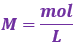Chemistry Practice Problems Weak Acid Strong Base Titrations Practice Problems Solution: A 250.0 mL solution of 0.100 M HClO is titrated wi...

🤓 Based on our data, we think this question is relevant for Professor Lewis' class at USF.

# Solution: A 250.0 mL solution of 0.100 M HClO is titrated with 0.200 M NaOH. What is the expected pH of the resulting solution once 50.0 mL of the NaOH solution has been added to the HClO solution?A. 4.68B. 7.36C. 7.71D. 8.20E. 13.30

###### Problem

A 250.0 mL solution of 0.100 M HClO is titrated with 0.200 M NaOH. What is the expected pH of the resulting solution once 50.0 mL of the NaOH solution has been added to the HClO solution?

A. 4.68

B. 7.36

C. 7.71

D. 8.20

E. 13.30

###### Solution

We are being asked to determine the pH of the HClO solution when 50.0 mL of NaOH solution is added to it.

We will calculate the pH of the HClO solution using the following steps:

Step 1. Calculate the initial amounts of HClO and NaOH in moles before the reaction happens.

Step 2. Write the chemical equation for the reaction between HClO and NaOH.

Step 3. Construct an ICF Chart.

Step 4. Calculate pH.

Step 1. Calculate the initial amounts of HClO and NaOH in moles before the reaction happens.

molarity (volume) → moles

Recall:*convert volumes from mL to L → 1 mL = 10-3 L

250 mL of 0.100 M HClOView Complete Written Solution

Weak Acid Strong Base Titrations

Weak Acid Strong Base Titrations

#### Q. Determine the pH of a solution created by mixing 127.6 mL of 0.278 M formic acid, HCO2H, with 148.3 mL of 0.329 M sodium hydroxide, NaOH.A. 1.32B. 10....

Solved • Fri Apr 28 2017 12:16:29 GMT-0400 (EDT)

Weak Acid Strong Base Titrations

#### Q. Calculate the pH at the equivalence point from the titration of 80.00 mL of 0.140 M hydrofluoric acid, HF, with 160.00 mL of 0.0700 M NaOH. Ka of HF i...

Solved • Wed Apr 26 2017 14:55:43 GMT-0400 (EDT)

Weak Acid Strong Base Titrations

#### Q. What is the pH of a solution that contains 1.0 L of 0.10 M CH3COOH and 0.080 M NaCH3COO after 0.03 moles of NaOH added? A. 4.33 B. 4.65 C. 4.74 D....

Solved • Thu Apr 20 2017 10:33:26 GMT-0400 (EDT)

Weak Acid Strong Base Titrations

#### Q. What would be the final pH if 0.0100 moles of solid NaOH were added to 100mL of a buffer solution containing 0.600 molar formic acid (ionization const...

Solved • Wed Apr 19 2017 11:40:14 GMT-0400 (EDT)